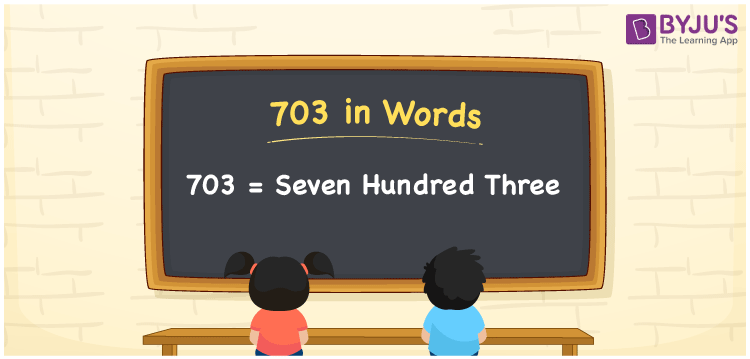# 703 in Words

703 in words is written as Seven hundred three. In both the International System of Numerals and the Indian System of Numerals, 703 is written as Seven hundred three. The number 703 is a Cardinal Number as it describes some quantity. For example, “there are 703 pages in that book”.

 703 in Words Seven hundred three Seven hundred three in Number 703

## 703 in English Words

We write 703 in English Words using the letters of the English alphabet. Therefore, we read 703 in English as “Seven hundred three.”## How to Write 703 in Words?

To write 703 in words, we shall use the place value chart. In the place value chart, write 3 in the ones, 0 in the tens, and 7 in the hundreds. Now let us make a place value chart to write the number 703 in words.

 Hundreds Tens Ones 7 0 3

Thus, we can write the expanded form as

7 × Hundred + 0 × Ten + 3 × One

= 7 × 100 + 0 × 10 + 3 × 1

= 700 + 0 + 3

= 703

= Seven hundred three.

703 is a natural number which is the successor of 702 and the predecessor of 704.

703 in words – Seven hundred three

• Is 703 an odd number? – Yes
• Is 703 an even number? – No
• Is 703 a perfect square number? – No
• Is 703 a perfect cube number? – No
• Is 703 a prime number? – No
• Is 703 a composite number? – Yes

## Frequently Asked Questions on 703 in Words

Q1

### How to write 703 in words?

703 in words is written as Seven hundred three.
Q2

### How to write 703 in words in the International and Indian System of Numerals?

In both the systems of numerals, 703 is written as Seven hundred three.
Q3

### How to write 703 in a place value chart?

In the place value chart, write 3 in ones, 0 in tens, and then 7 in the hundreds place.